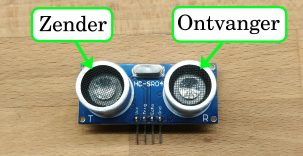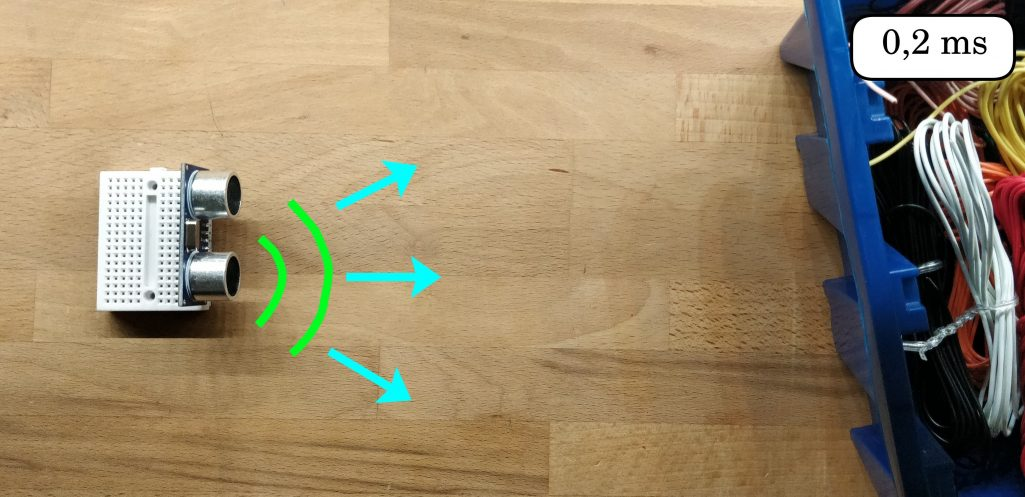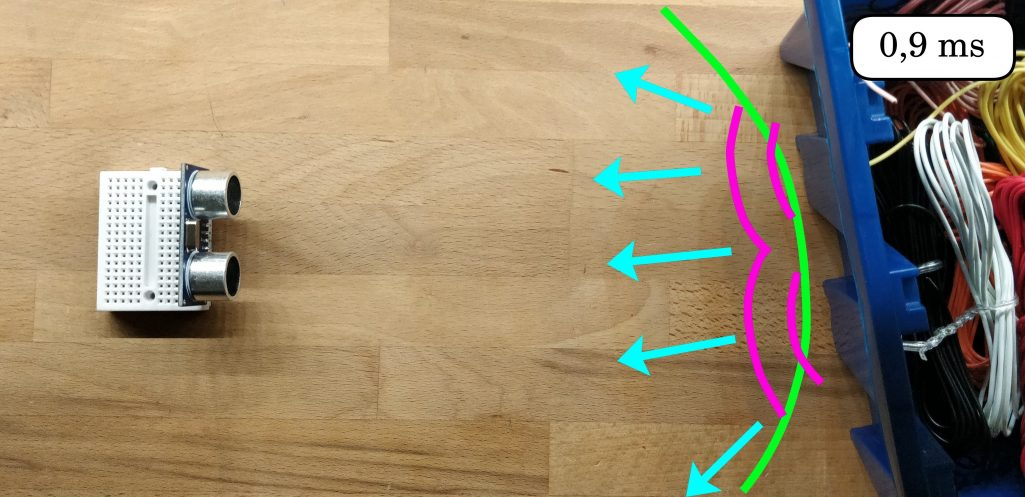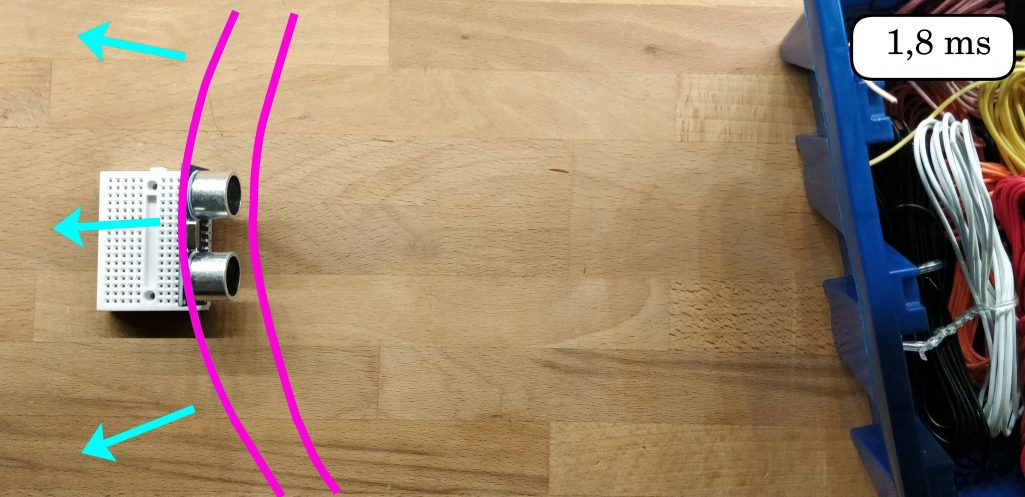# Component – Ultrasonic distance sensor

14 juni 2020 components

The ultrasonic sensor is a simple, but incredibly powerful sensor. It uses ultrasonic sound to measure distance.

# The measuring process

So an ultrasonic sensor uses ultrasonic sound to measure distance. But how does this work exactly?

(Image translation – Sender=Zender – Receiver= Ontvanger)An ultrasonic sensor module contains both a transmitter and a receiver. These are the two parts that look like eyes. Next to the transmitter is a T, coming from the word transmitter. The receiver has an R of the word receiver.When we start a measurement, ultrasonic waves are emitted from the transmitter. The frequency of this sound is so high that people can no longer hear it. Moreover, the volume is also quite low.The sound waves will move through space at the speed of sound. Until something gets in the way. When these waves encounter an object, they’re reflected back. The waves will now move back in the direction of the sensor.The sound waves will return to our sensor after a while. The waves will propagate even further. But at this moment the receiver recognizes that the sound waves have returned.

We see that, in the measurement in the pictures, it took 1.8 milliseconds until the sound waves came back again. Because sound waves propagate at a certain speed, the speed of sound, we can use this data to calculate the distance of the object.

## The calculation

At room temperature, the sound velocity is 343 meters per second (ms). So every second a sound wave will travel 343 meters.

A millisecond is a thousandth of a second. In a millisecond, a sound wave will also travel a thousandth of 343 meters. This is 0.343 meters or 34.3 centimeters.

In the measurement above, we see that it took 1.8 milliseconds for the sound to go to an object, and come back again. The distance covered by the sound waves is therefore ‘1.8 milliseconds times 34.3 centimeters’ 61.7 centimeters.

The sound waves have therefore traveled a total of 61.7 centimeters. The object will be exactly at the halfway point of this traveled route, this is because the route towards the object is as long as the route away from the object, back to the sensor. So the object is at a distance of ‘61.7 parts by 2 is’ 30.9 centimeters.

No measurement

It sometimes happens that sound waves are emitted that never come back. This may be because of the sound waves:

1. are not reflected back in the direction of the sensor.
2. on the way, no object is encountered and therefore not reflected at all.
3.  traveled such a long distance that the amplitude (the volume) is so small that the sound waves are no longer recognized by the receiver.

In these cases, depending on the type, the ultrasonic sensor will never give the signal that a sound wave has been received, or after a certain time, it will still pretend that the module has recognized a sound wave. In the latter case, it will seem as if a very large distance has been measured. The HC-SR04, the module explained below, uses the last method.

## The  HC-SR04

Among hobbyists, the most commonly used ultrasonic sensor is the HC-SR04. This is because it is very cheap and easy to use the module. Also in our workshop, we mainly use this type of sensor. For this module the following datasheet is available.

## Pinout

The HC-SR04 has four pins. These are VCC, GND, Trigger, and Echo.

To power the module, the VCC and GND will need to be connected to a 5-volt power source. This may be an Arduino.

To start a measurement, we can send a short signal over the trigger pin. We set the trigger pin to 5 volts for at least 10 microseconds. When the ultrasonic waves are emitted, the module sets the echo pin to 5 volts. When the ultrasonic waves are received again, we set the echo pin back to 0 volts (ground). The whole process will look like this on an oscilloscope:

# With the Arduino

To work with the HC-SR04 sensor we generally use the ‘HCSR04’ library in our workshop by ‘Martin Sosic’. However, you don’t have to use a library, the lab is still developing one. But it does make writing code a lot easier.

The HCSR04 library contains the following example code:

```#include &amp;amp;amp;lt;HCSR04.h&amp;amp;amp;gt;

UltraSonicDistanceSensor distanceSensor(13, 12); // Initialize the sensor with the triger pin on D13 and the echo pin on D12.
void setup () {
Serial.begin(9600); // Initialize Serial so that we can use the serial monitor on the computer}

void loop () {
Serial.println(distanceSensor.measureDistanceCm()); // Doe een meting, en stuur deze door naar de seriële monitor
delay(500); // wait 500 milliseconds = 0,5 seconds
}

```

In order to understand how this code works, we divide it into pieces.

`#include &amp;amp;amp;lt;HCSR04.h&amp;amp;amp;gt;`

With this code we indicate that we want to use the HCSR04 library. This ensures that Arduino will load this library.

`UltraSonicDistanceSensor distanceSensor(13, 12);`

We indicate that we want to use an ultrasonic sensor with the trigger pin D13 and the echo pin D12. We give this sensor the name ‘distanceSensor’, this should also be different if it starts with a letter. We could also change the pins we want to use, we could change 12 and 13 to any other pin, to use the analog pins we fill in for example A0 or A1.

`distanceSensor.measureDistanceCm();`

With the code above we start a measurement on the module called distanceSensor, the distance will be given in centimeters.

But what do we do with the result of this measurement?

`double afstand = distanceSensor.measureDistanceCm();`

We could put the result in a variable, so we can use this number later in the code. We can do this with the code above.

Or we can use an if-statement to execute our code only when the distance is greater than for example 50 centimeters:

```if(distanceSensor.measureDistanceCm() &amp;amp;amp;gt; 50){
// The code we put here will be executed if the measured distance is greater than 50 centimetres.
}else{
// if the distance is less than 50 centimeters, the code we put here will be executed }

```

# Next step

Now that you know how the ultrasonic sensor works, you can use it in your projects. Don’t you have a project yet? No problem, we also have labs you can follow! For example, follow the lab ‘Arduino afstand Smiley’.